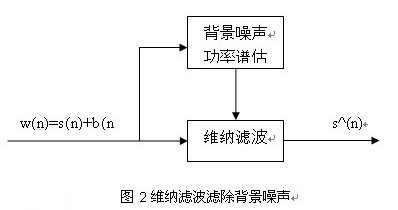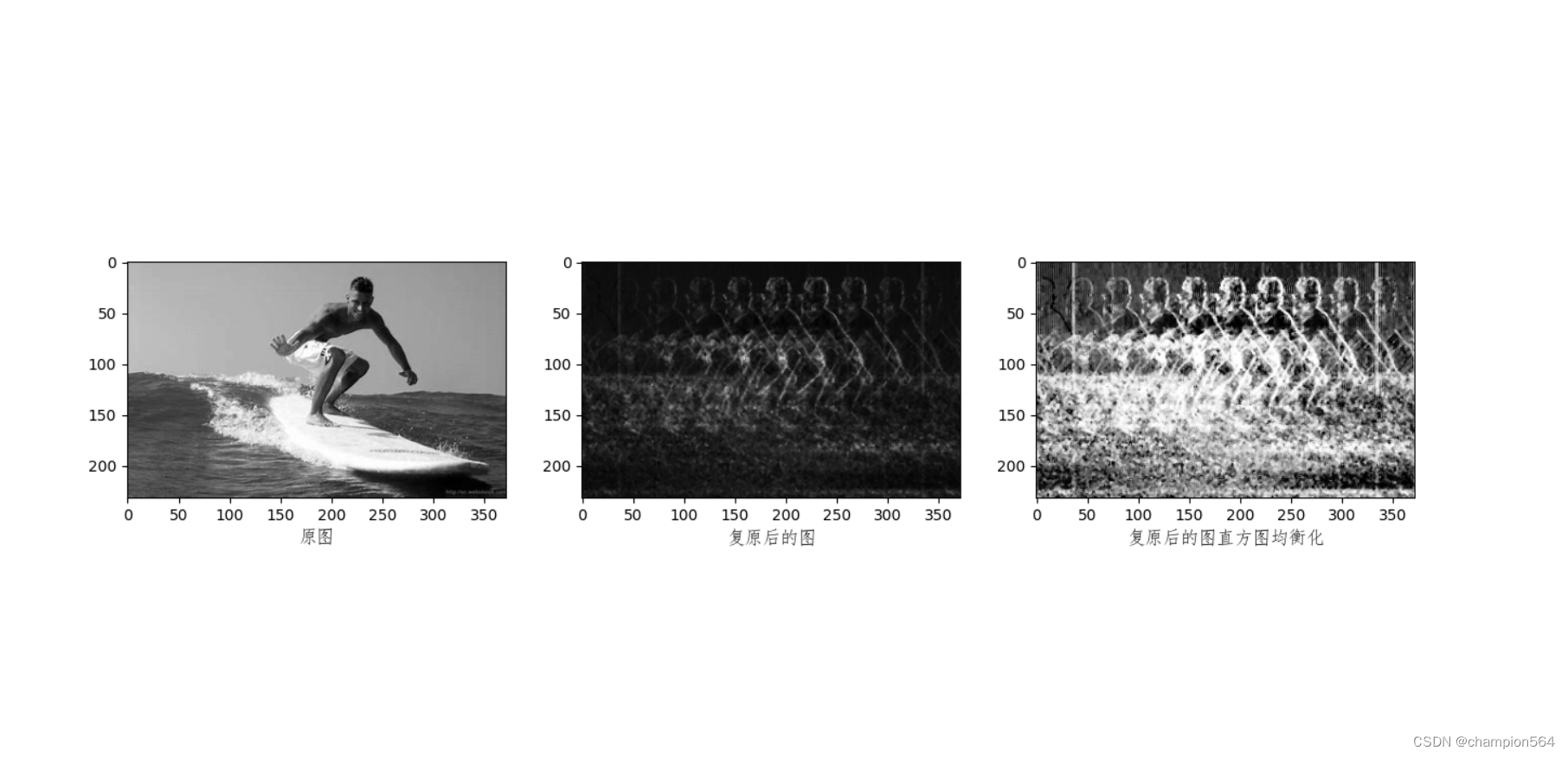> 文档中心 > 图像处理之维纳滤波还原图像、直方图均值化【附代码、亲测有效】

# 图像处理之维纳滤波还原图像、直方图均值化【附代码、亲测有效】

• 一、基础知识
• 二、效果图
• 三、代码

## 一、基础知识（1）首先假设点扩散函数为高斯模型 ，加性白噪声为高斯噪声 ，理想的未退化的图像为 ，那么退化图像可表示为 ，要想恢复出理想图像，就要估计点扩散函数 和加性白噪声 ；
（2）为了更好地估计叠加有白噪声的高斯模糊图像的点扩展函数，可以先对图像进行去噪处理。因此我们采用均值滤波对退化图像进行去噪；
（3）采用最大似然估计算法来估计退化图像的点扩展函数 ，即寻找最适合的点扩展函数来极大化似然函数，先假设一个初始值，然后通过迭代方式估计出点扩展函数；
（4）估计加性白噪声 ，采用matlab图像处理工具箱中的二维维纳滤波函数来估计噪声参数；
（5）最后采用维纳滤波对退化图像进行复原。维纳滤波也称最小均方误差滤波，它能处理被退化函数退化和噪声污染的图像。该滤波方法建立在图像和噪声都是随机变量的基础之上，目标是找到理想图像 的一个估计 ，使它们之间的均方误差最小，即 。

（1）均值滤波去噪
（2）估计加性白噪声模型参数
（3）估计点扩散函数
（4）维纳滤波进行图像复原

## 二、效果图## 三、代码

``# 利用维纳滤波还原图像import cv2import numpy as npfrom numpy import fftfrom matplotlib import pyplot as pltimport cmathdef degradation_function(m, n,a,b,T):    P = m / 2 + 1    Q = n / 2 + 1    Mo = np.zeros((m, n), dtype=complex)    for u in range(m): for v in range(n):     temp = cmath.pi * ((u - P) * a + (v - Q) * b)     if temp == 0:  Mo[u, v] = T     else:  Mo[u, v] = T * cmath.sin(temp) / temp * cmath.exp(- 1j * temp)    return Modef image_mapping(image):    img = image/np.max(image)*255    return imgif __name__ == '__main__':    img = cv2.imread('C:/Users/admin/Desktop/OIP-C.jpg',0)    m, n = img.shape    a = 1e-6    b = 0.1    T = 0.1    K = 1e-11    G = fft.fft2(img)    G_shift = fft.fftshift(G)    H = degradation_function(m, n,a,b,T)    F = G_shift *((np.abs(H)*np.abs(H)) / (H*np.abs(H)*np.abs(H)+K))    f_pic = np.abs(fft.ifft2(F))    res = image_mapping(f_pic)    res1 = res.astype('uint8')    res1 = cv2.medianBlur(res1, 3)    res2 = cv2.equalizeHist(res1)    plt.subplot(131)    plt.imshow(img,cmap='gray')    plt.xlabel('原图', fontproperties='FangSong', fontsize=12)    plt.subplot(132)    plt.imshow(res1,cmap='gray')    plt.xlabel('复原后的图', fontproperties='FangSong', fontsize=12)    plt.subplot(133)    plt.imshow(res2,cmap='gray')    plt.xlabel('复原后的图直方图均衡化', fontproperties='FangSong', fontsize=12)    plt.show()``